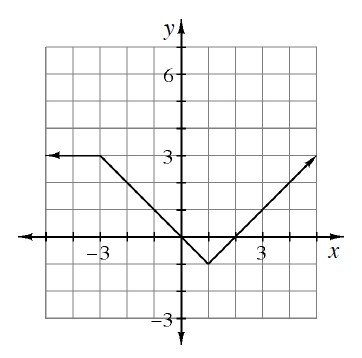### Home > PC > Chapter 3 > Lesson 3.2.3 > Problem3-93

3-93.

Given the graph of $y=f(x)$ below, sketch the graph for each of the following transformations.1. $2f(x)$

What happens to the graph if all of the outputs are multiplied by $2$?

1. $f(2x)$

If $x=2$, then on the transformed graph $f(2)$ is equivalent to the original $f(4)$.
Or, if the original graph was $y=x−2$, the transformed graph would be $y=2x−2$.

1. $-f(x)$

Reflect the curve across the $x$-axis because this transformation makes all of the outputs the negative of the original function.

1. $f(-x)$

Reflect the curve across the $y$-axis because this transformation makes all of the inputs the opposite of the original function.

Use the eTool below to graph the functions.
Click the link at right for the full version of the eTool:  3-93 eTool